# Chapter 6 Momentum 1 MOMENTUM Momentum inertia in

• Slides: 36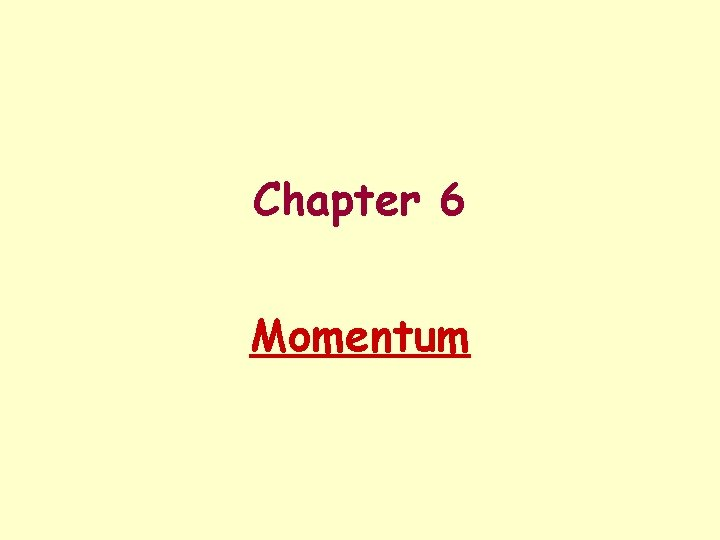Chapter 6 Momentum1. MOMENTUM • Momentum - inertia in motion • Momentum = mass times velocity Units - kg m/s or sl ft/s2. IMPULSE • Collisions involve forces (there is a Dv). • Impulse = force times time. Units - N s or lb s3. IMPULSE CHANGES MOMENTUM Impulse = change in momentum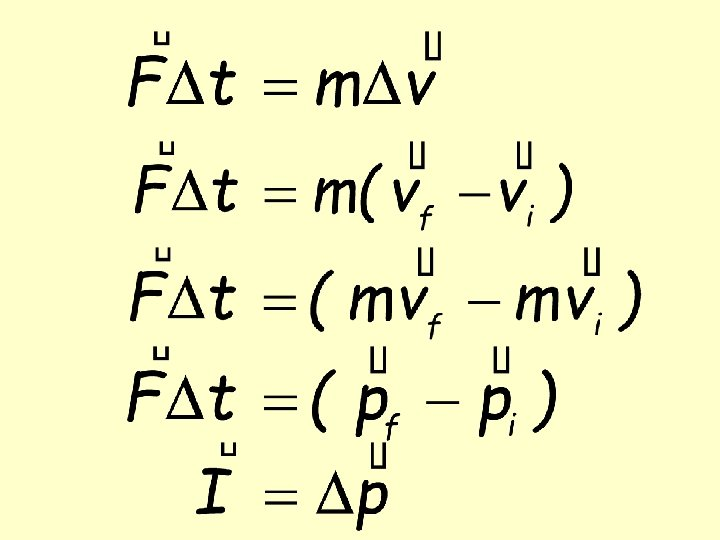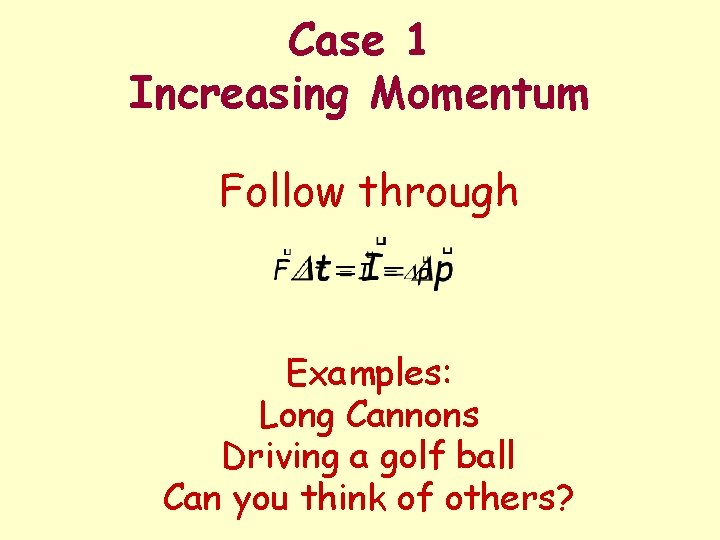Case 1 Increasing Momentum Follow through Examples: Long Cannons Driving a golf ball Can you think of others?Video Clip Tennis racquet and ball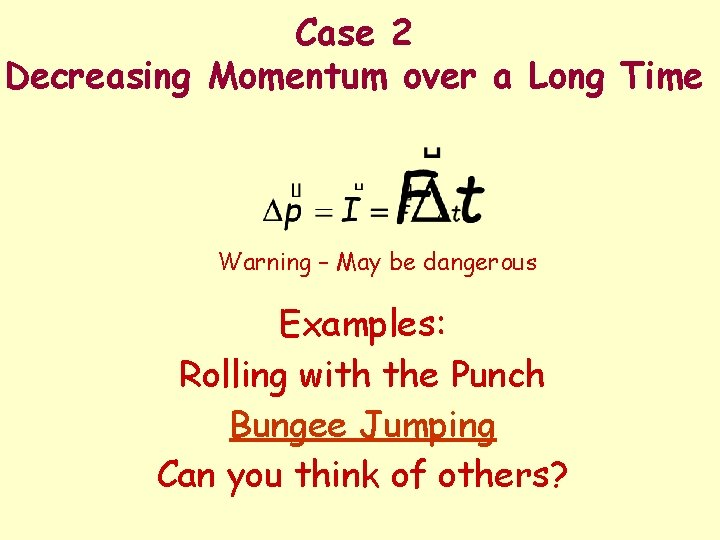Case 2 Decreasing Momentum over a Long Time Warning – May be dangerous Examples: Rolling with the Punch Bungee Jumping Can you think of others?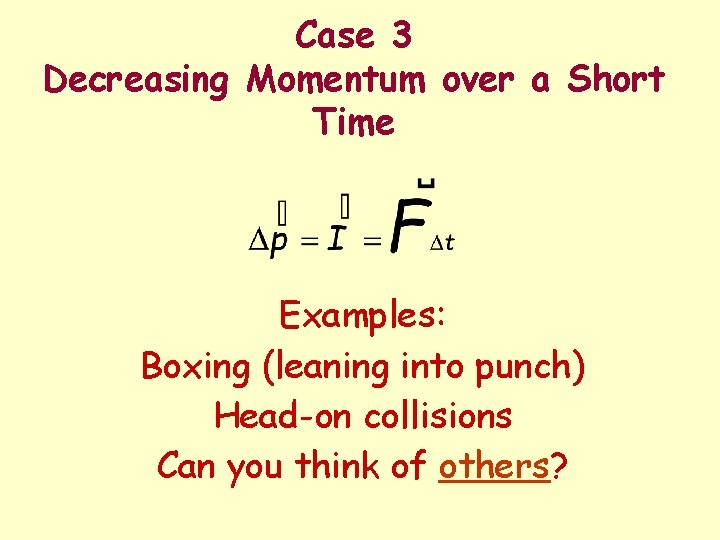Case 3 Decreasing Momentum over a Short Time Examples: Boxing (leaning into punch) Head-on collisions Can you think of others?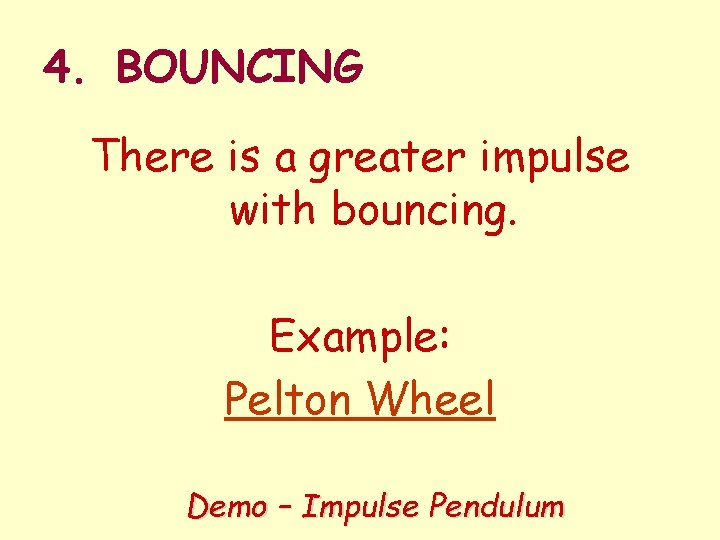4. BOUNCING There is a greater impulse with bouncing. Example: Pelton Wheel Demo – Impulse Pendulum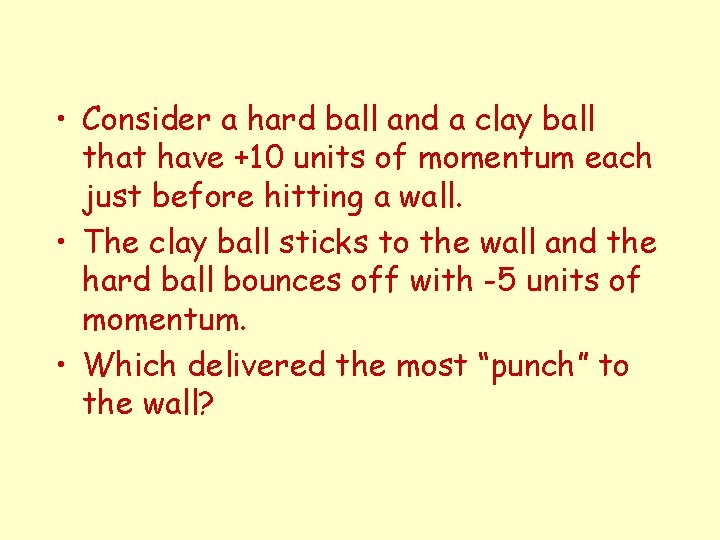• Consider a hard ball and a clay ball that have +10 units of momentum each just before hitting a wall. • The clay ball sticks to the wall and the hard ball bounces off with -5 units of momentum. • Which delivered the most “punch” to the wall?Initial momentum of the clay ball is 10. Final momentum of clay ball is 0. The change is 0 - 10 = - 10. It received - 10 impulse so it applied + 10 to the wall.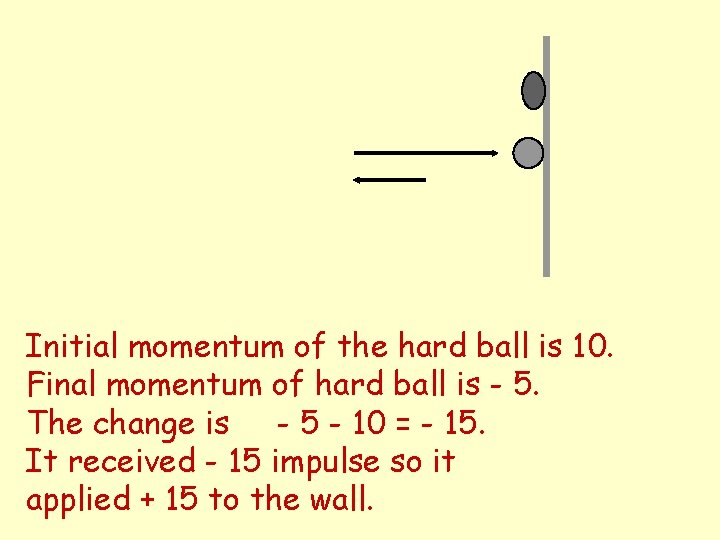Initial momentum of the hard ball is 10. Final momentum of hard ball is - 5. The change is - 5 - 10 = - 15. It received - 15 impulse so it applied + 15 to the wall.5. CONSERVATION OF MOMENTUM Example: Rifle and bullet Demo - Rocket balloons (several) Demo - Clackers Video - Cannon recoil Video - Rocket scooterConsider two objects, 1 and 2, and assume that no external forces are acting on the system composed of these two particles. Impulse applied to object 1 Impulse applied to object 2 Apply Newton’s Third Law Total impulse applied to system or• Internal forces cannot cause a change in momentum of the system. • For conservation of momentum, the external forces must be zero.Chapter 6 Review Questions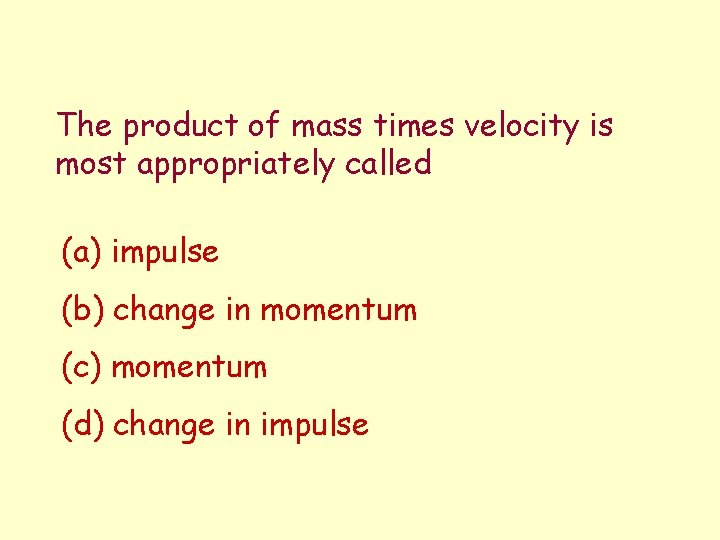The product of mass times velocity is most appropriately called (a) impulse (b) change in momentum (c) momentum (d) change in impulseYou jump off a table. When you land on the floor you bend your knees during the landing in order to (a) make smaller the impulse applied to you by the floor (b) make smaller the force applied to you by the floor (c) both (a) and (b)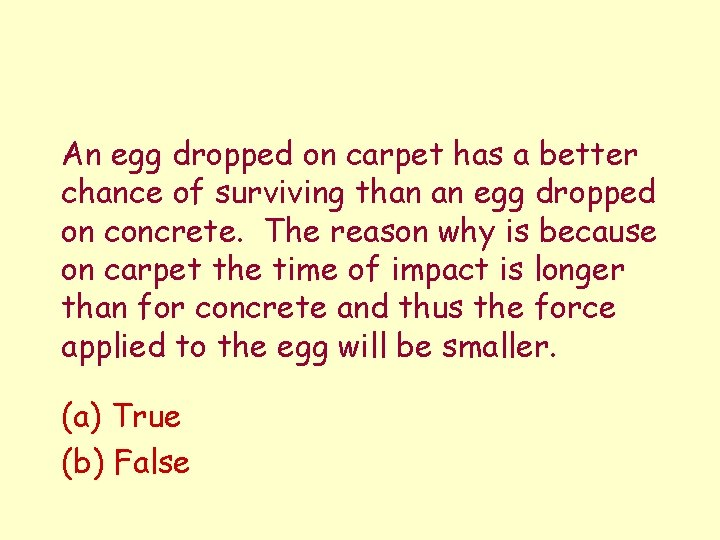An egg dropped on carpet has a better chance of surviving than an egg dropped on concrete. The reason why is because on carpet the time of impact is longer than for concrete and thus the force applied to the egg will be smaller. (a) True (b) False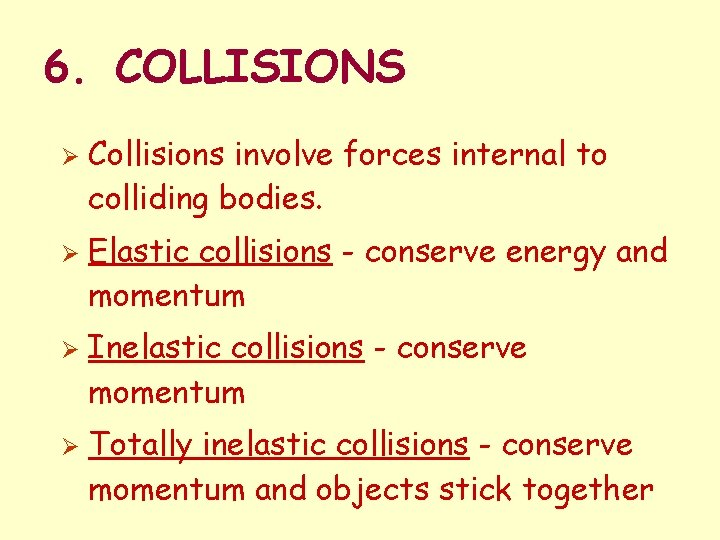6. COLLISIONS Ø Ø Collisions involve forces internal to colliding bodies. Elastic collisions - conserve energy and momentum Inelastic collisions - conserve momentum Totally inelastic collisions - conserve momentum and objects stick togetherDemos and Videos Terms in parentheses represent what is conserved. ØDemo – Air track collisions (momentum & energy) ØDemo - Momentum balls (momentum & energy) ØDemo - Hovering disks (momentum & energy) ØDemo - Small ball/large ball drop ØDemo - Funny Balls ØVideo - Two Colliding Autos (momentum)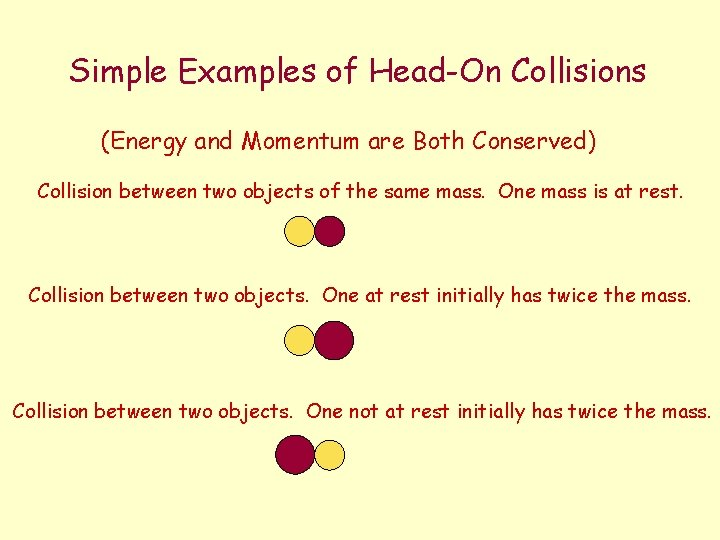Simple Examples of Head-On Collisions (Energy and Momentum are Both Conserved) Collision between two objects of the same mass. One mass is at rest. Collision between two objects. One at rest initially has twice the mass. Collision between two objects. One not at rest initially has twice the mass.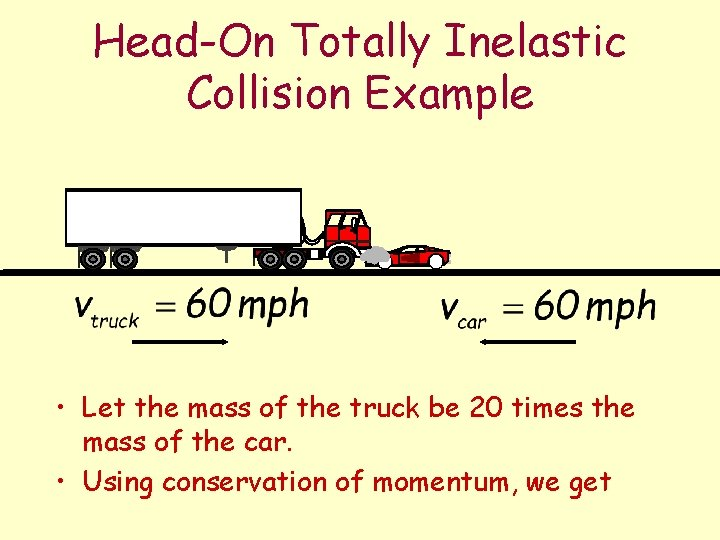Head-On Totally Inelastic Collision Example • Let the mass of the truck be 20 times the mass of the car. • Using conservation of momentum, we getinitial momentum of system = final momentum of system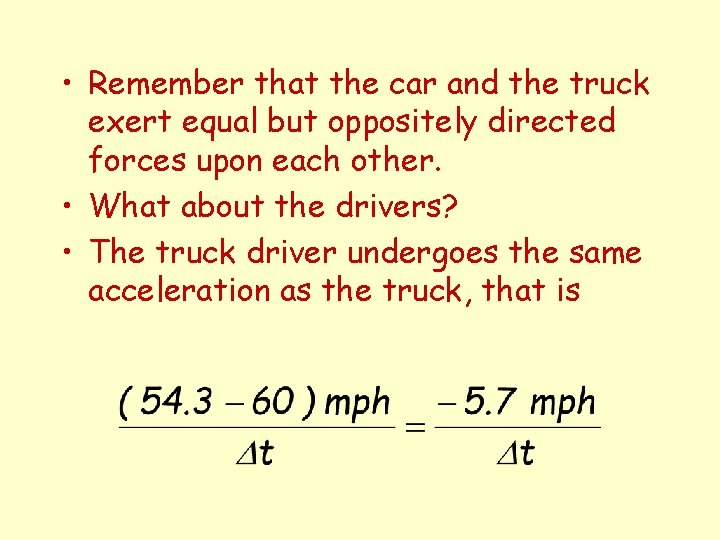• Remember that the car and the truck exert equal but oppositely directed forces upon each other. • What about the drivers? • The truck driver undergoes the same acceleration as the truck, that is• The car driver undergoes the same acceleration as the car, that is The ratio of the magnitudes of these two accelerations is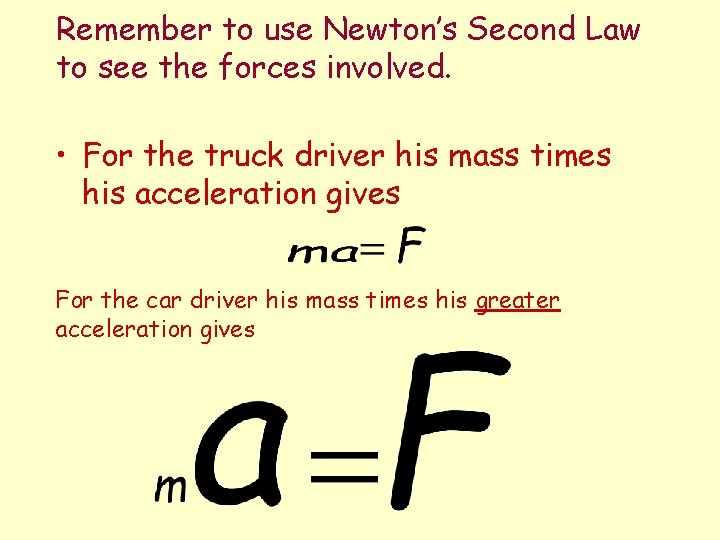Remember to use Newton’s Second Law to see the forces involved. • For the truck driver his mass times his acceleration gives For the car driver his mass times his greater acceleration gives, big trucks that is. • Don’t mess with TRUCKS T • Your danger is of the order of twenty times greater than that of the truck driver.7. More Complicated Collisions Vector Addition of Momentum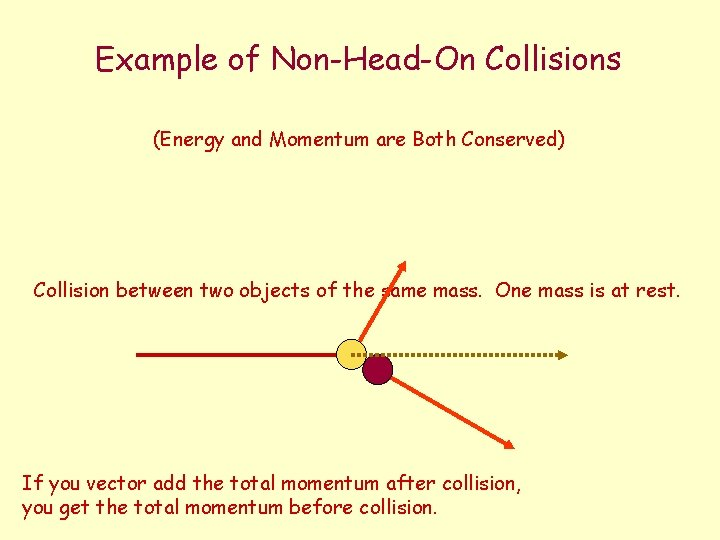Example of Non-Head-On Collisions (Energy and Momentum are Both Conserved) Collision between two objects of the same mass. One mass is at rest. If you vector add the total momentum after collision, you get the total momentum before collision.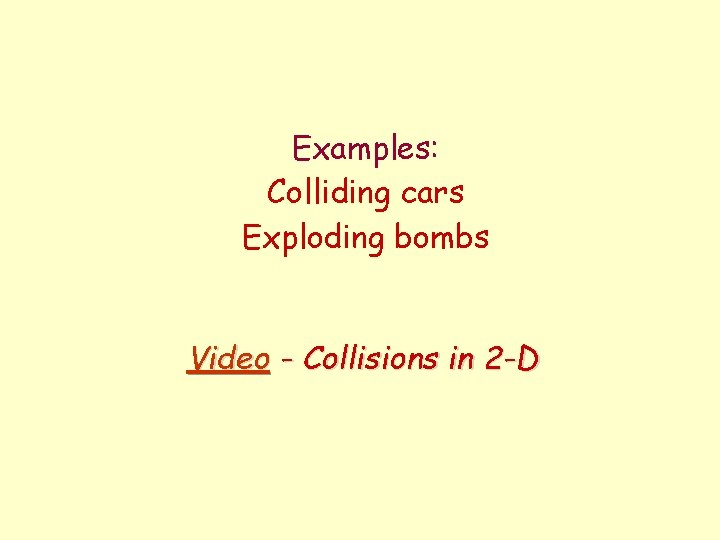Examples: Colliding cars Exploding bombs Video - Collisions in 2 -DChapter 6 Review QuestionsIn which type of collision is energy conserved? (a) elastic (b) inelastic (c) totally inelastic (d) All of the above (e) None of the above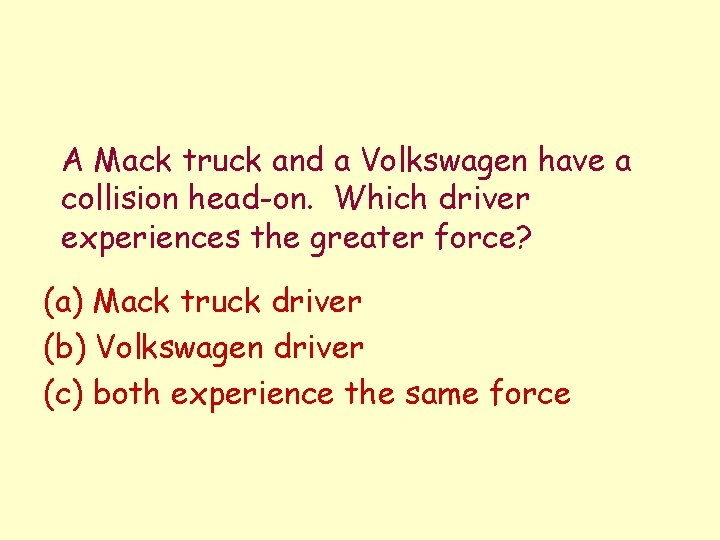A Mack truck and a Volkswagen have a collision head-on. Which driver experiences the greater force? (a) Mack truck driver (b) Volkswagen driver (c) both experience the same force